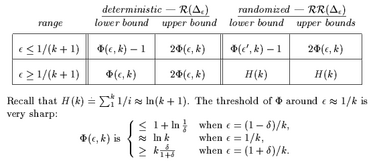## neal young / Young00Online

• Journal of Algorithms 37(1):218-235(2000); SODA'98Elias Koutsoupias and Christos Papadimitriou introduced the so-called diffuse-adversary model for analyzing paging strategies such as least-recently-used (LRU) . Briefly, the diffuse adversary model is as follows. Fix some $$\epsilon >0$$ Run the algorithm, and, for each request, allow the adversary to assign a probability distribution to the items such that no item gets probability more than $$\epsilon$$, then request an item randomly from the distribution. (When $$\epsilon$$ is near 1, one has the standard competitive-analysis model; when $$\epsilon$$ is near 0, the adversary is severely handicapped.) The algorithm is $$c$$-competitive if the expected cost of the algorithm on the adversarial sequence is at most $$c$$ times the expected cost of the optimal algorithm.

Koutsoupias and Papadimitriou proved that LRU was optimally competitive (among deterministic on-line algorithms) according to the model, but left open the question of what the performance guarantee for LRU actually was. This paper answers the question, giving an explicit formula for the performance guarantee within a factor of two. The paper also shows that the performance guarantee of first-in-first-out (FIFO) is much worse than that of LRU, and analyzes the performance guarantees of randomized on-line paging strategies.
Journal version of Bounding the diffuse adversary.